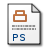# Parameter-free H(div) preconditioning for mixed finite element formulation of diffusion problems

Powell, Catherine (2005) Parameter-free H(div) preconditioning for mixed finite element formulation of diffusion problems. IMA Journal of Numerical Analysis, 25 (4). pp. 783-796. ISSN 1464-3642Postscript narep453.ps Download (1MB)

## Abstract

Mixed finite element formulations of generalised diffusion problems yield linear systems with ill-conditioned, symmetric and indefinite coefficient matrices. Preconditioners with optimal work complexity that do not rely on artificial parameters are essential. We implement lowest order Raviart–Thomas elements and analyse practical issues associated with so-called ‘H(div) preconditioning’. Properties of the exact scheme are discussed in Powell & Silvester (2003, SIAM J. Matrix Anal. Appl., 25, 718–738). We extend the discussion, here, to practical implementation, the components of which are any available multilevel solver for a weighted H(div) operator and a pressure mass matrix. A new bound is established for the eigenvalue spectrum of the preconditioned system matrix and extensive numerical results are presented.

Item Type: Article H(div) preconditioning, mixed finite elements, saddle-point problems, multigrid, preconditioning MSC 2010, the AMS's Mathematics Subject Classification > 15 Linear and multilinear algebra; matrix theoryMSC 2010, the AMS's Mathematics Subject Classification > 65 Numerical analysis Dr C.E. Powell 23 May 2006 20 Oct 2017 14:12 http://eprints.maths.manchester.ac.uk/id/eprint/287View Item